# Homework H1.I - Sp23

 Problem statement Solution videoAsk your questions here. Or, if you can help someone by answering their questions, then do that here. You can learn either way.

DISCUSSION
This is a standard relative motion type of question. You are given complete information on the velocity vectors for A and P (both x- and y-components). And, you are asked to find the velocity of P with respect to A.

To do so, use the basic equation for relative velocity between two points:  vP/A = vP - vA. From this result, you are then asked to find the angle of the observed velocity of P, with this last step being just trig.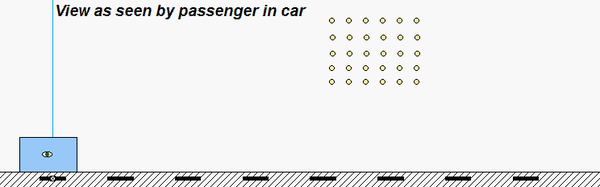# Homework H1.J - Sp23

 Problem statement Solution video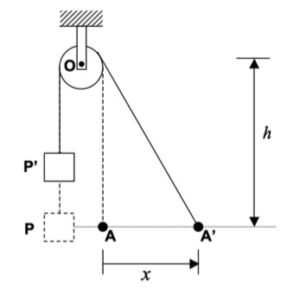Ask your questions here. Or, if you can help someone by answering their questions, then do that here. You can learn either way.

DISCUSSION
This is a standard cable/pulley kinematics problem. You need to write the length, L, of the cable in terms of motion parameters for A and P. Let sP represent the distance that P is below the center of the pulley. From this, you can write: L = sP + sqrt(x^2 + h^2) + constant. Setting dL/dt = 0 gives you the relationship between sP_dot and x_dot.From the animation above, you can see that the speed of crate is never any greater than the speed of the worker, A. Can you see this result in your work?

# Homework H1.G - Sp23

 Problem statement Solution video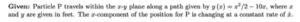You can ask questions or answer questions of others here. You can learn from either.

DISCUSSION
Shown in the animation below is the path taken by Particle P.

• As expected the unit vectors et and en are tangent and normal to the path of P, repectively.
• Also seen there is that the velocity vector is always aligned with the tangent unit vector, et, since v = v et.
• The acceleration vector, in general, has both tangential and normal components of acceleration. The normal component always points in the same direction as the unit normal vector, en. The acceleration vector either points "forward" of the motion (for which the rate of change of speed is positive; i.e., increasing is speed), or points "backward" of the motion (for which the rate of change of speed is negative; i.e., slowing down).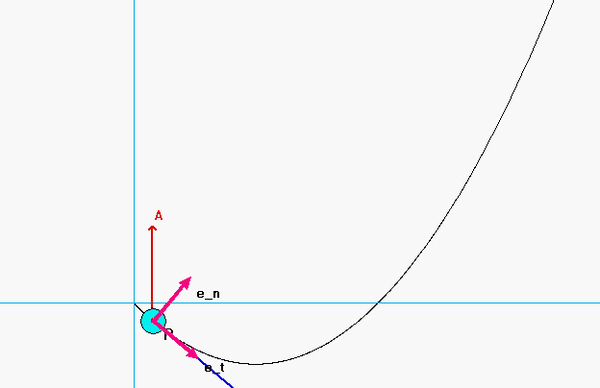HINTS
Recall that since you do not know the y-component of motion of P explicitly in terms of time, you will need to use the chain rule to find dy/dt.

It is most convenient to determine the rate of change of speed through the vector projection of the acceleration vector, a, onto the unit tangent vector, et. How do you find the tangent unit vector? Simply divide the velocity vector by its magnitude!   etv/v .

Once you know the rate of change of speed, you can find the radius of curvature ρ through the magnitude of the acceleration.

# Homework H1.H - Sp23

 Problem statement Solution videoDISCUSSION
This problem involves the joint descriptions of Cartesian and polar coordinates: Cartesian coordinates are used to describe the path of P, and the polar description is to be used since r_dot = -vA for the motion of P.

As given in the problem statement, you are to first use the Cartesian description to derive the velocity and acceleration vectors for P. Since y = y(x), you will need to use the chain rule of differentiation to find y_dot:  y_dot = (dy/dx)*x_dot = (-4x)*x_dot. Taking an additional derivative gives: y_ddot = -4*x_dot^2 - 4*x*x_ddot. Using these in the Cartesian description gives v and a in terms of x_dot and x_ddot.

Now draw in the unit vectors of er and eθ: For θ = 0, we see that i = er and j = eθ. We use this to find x_dot and θ_dot from the velocity equation, and x_ddot from the acceleration equation.

.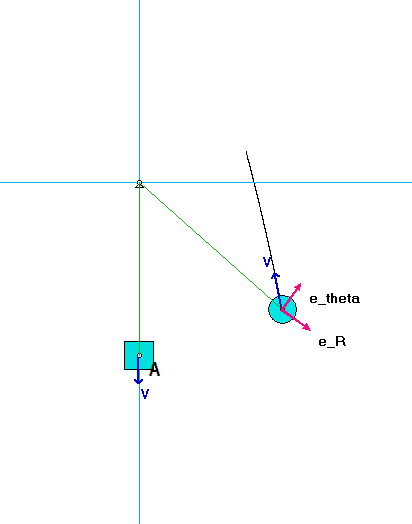Ask your questions here. Or, if you can help someone by answering their questions, then do that here. You can learn either way.

# Homework H1.E - Sp23

 Problem statement Solution video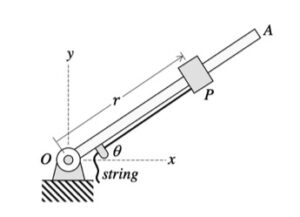DiscussionAs you watch particle P move through its path, focus on a few things:

• Direction of the velocity vector. The velocity of the ball is always tangent to the path, as expected. Note that the unit tangent vector e_t points in the same direction as the velocity.
• Direction of the acceleration vector. The acceleration of the P always points "inward" on the path, again, as expected. Here you see that the acceleration vector points in the positive e_t direction; therefore, its speed must be increasing with time (as it is).
• Directions of the polar unit vectors. The e_r unit vector always points outward from  point O toward P. The e_θ unit vector is perpendicular to e_r, and in the direction of increasing θ, as seen in the animation above.

Your task is to calculate R and θ, and their time derivatives. Here, you are given R and θ explicitly as a function of time. Therefore, the chain rule of differentiation is not needed.

# Homework H1.F - Sp23

 Problem statement Solution video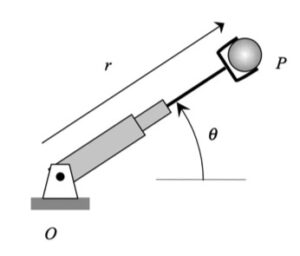Discusssion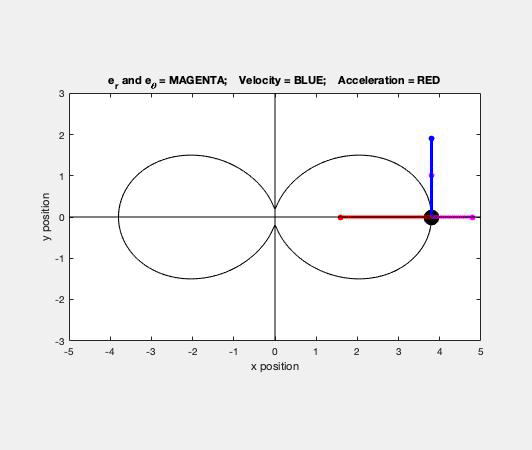As you watch the sphere move through its path, focus on a few things:

• Direction of the velocity vector. The velocity of the ball is always tangent to the path, as expected. Note that the unit tangent vector e_t points in the same direction as the velocity.
• Direction of the acceleration vector. The acceleration of the ball always points "inward" on the path, again, as expected. When P is slowing down, you see that the acceleration vector points in the negative e_t direction. Similarly, when P is increasing in speed, the acceleration vector points in the positive e_t direction.
• Directions of the polar unit vectors. The e_r unit vector always points outward from  point O toward the sphere. The e_θ unit vector is perpendicular to e_r, and in the direction of increasing θ, as seen in the animation above.

Your task is to calculate R, R_dot and R_ddot using the given equation relating R and θ. This will require derivatives with respect to TIME; however, since R is given in terms of θ, you will need to use the chain rule of differentiation. For example: R_dot = dR/dt = (dR/dθ)(dθ/dt).

# Homework H1.C - Sp23

[/embed]

 Problem statement Solution video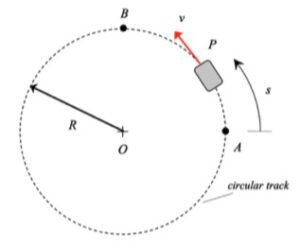DISCUSSION and HINTS

As expected, the acceleration of P has both non-zero tangential and normal components.

• From the equation provided for speed as a function of distance traveled, the speed of P is monotonically decreasing over the range of motion shown in the animation below. Therefore, the tangential component of acceleration always points "backward" of the direction of motion.
• The normal component decreases as P moves along the track since the speed of P is decreasing.

Can you see these two things in the animation below?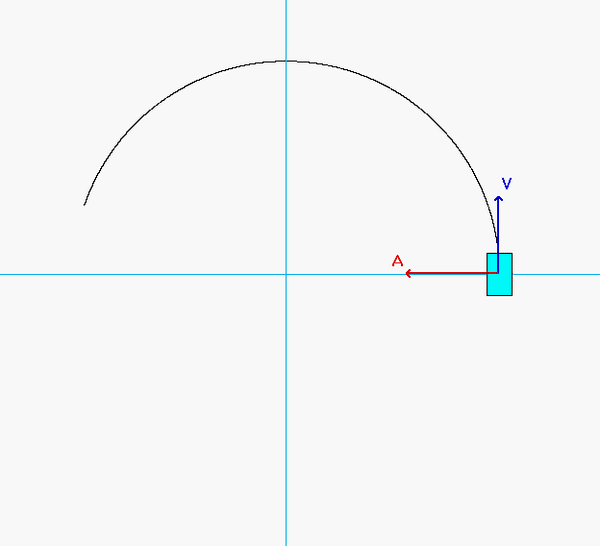Recall that the general path description velocity and acceleration equations are given by the following:

v = v*et
a = v_dot*et + (v2/ρ)*en

Note that v_dot = dv/dt. For this problem, we do NOT know the speed as a function of time; instead, we know speed as a function of position, s. To find v_dot, we need to use the chain rule of differentiation:  dv/dt = (dv/ds)*(ds/dt) = v*(dv/ds).

# Homework H1.D - Sp23

 Problem statement Solution video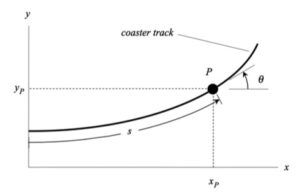DISCUSSION:

# Homework H1.A - Sp23

 Problem statement Solution videoDiscussion

Consider the animation below of the motion for particle P:

• For all time, P moves with a positive x-component and a negative y-component of both velocity and acceleration. Why? Can you see this in the animation?
• As we will see in the next class period when we talk about the "path description" of kinematics, the velocity of a point is always tangent to the path of the point, and the acceleration always points "inward" on the path of the point. Do you see this in the animation?The components of velocity and acceleration of P are found directly from the time derivatives of the position components:

vP = x_dot i + y_dot j
aP =
x_ddot i + y_ddot j

# Homework H1.B - Sp23

 Problem statement Solution video

Given: A particle P travels on a path described by the Cartesian coordinates of y = cx(b-x). The x-component of velocity for P is a constant.

From the animation below, you see that the velocity of P is always tangent to the path, and that the acceleration always points inward of the path. In the next class when we discuss the path description of kinematics, we will discover that this is true in general.

Note below that in this case the acceleration of P is always vertical. Do you know why?HINTS:
The path of P is not given in terms of x- and y-coordinates that are known explicit functions of time (instead, the y-coordinate depends explicitly on the x-coordinate). For velocity and acceleration, we need the derivatives of x and y with respect to time. How do we do this? Recall the chain rule of differentiation:

dy/dt = (dy/dx)*(dx/dt)

Use this in setting up the components of velocity and acceleration.